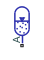# Gas-Charged Accumulator (IL)

Gas-charged accumulator in an isothermal liquid network

Since R2020a

•Libraries:
Simscape / Fluids / Isothermal Liquid / Tanks & Accumulators

## Description

The Gas-Charged Accumulator (IL) block represents a gas-charged accumulator in an isothermal liquid network. The accumulator consists of a precharged gas chamber and a liquid chamber. The chambers are separated by a bladder, a piston, or any kind of a diaphragm.

As the liquid pressure at the accumulator inlet becomes greater than the precharge pressure, liquid enters the accumulator and compresses the gas through a polytropic process. A decrease in the liquid pressure causes the gas to decompress and discharge stored liquid into the system. The separator motion is restricted by a hard stop when the liquid volume is zero and when the liquid volume is at the liquid chamber capacity. The liquid chamber capacity is the total accumulator volume minus the minimum gas volume.

Inlet liquid resistance, and separator properties such as inertia and damping are not modeled. The flow rate is positive if liquid flows into the accumulator.This diagram represents a gas-charged accumulator. The total accumulator volume, `VT`, is divided into the liquid chamber on the left and the gas chamber on the right by the vertical separator. The distance between the left side and the separator defines the liquid volume, `VL`. The distance between the right side and the separator defines the gas volume, `VG`. The liquid chamber capacity, `VC`, is less than the total accumulator volume, so that the gas volume never becomes zero:

`$\begin{array}{l}{V}_{L}={V}_{T}-{V}_{G}\\ {V}_{C}={V}_{T}-{V}_{dead}\end{array}$`

where:

• VT is the total volume of the accumulator, including the liquid chamber and the gas chamber.

• VL is the volume of the liquid in the accumulator.

• VG is the volume of the gas in the accumulator.

• VC is the liquid chamber capacity (`VT` - `VG`).

• Vdead is the gas chamber dead volume, a small portion of the gas chamber that remains filled with gas when the liquid chamber is at capacity.

The hard stop contact pressure is modeled with a stiffness term and a damping term. The relationship of the gas pressure and gas volume between the current state and the precharge state is polytropic and pressure is balanced at the separator:

`${p}_{G}{V}_{G}^{{k}_{sh}}={p}_{pr}{V}_{T}^{{k}_{sh}},$`

where:

• pG is the gas pressure in the gas chamber.

• ppr is the pressure in the gas chamber when the liquid chamber is empty

• ksh is the specific heat ratio (adiabatic index).

### Conservation of Mass

Conservation of mass is represented by the following equation.

where:

• pI is the liquid pressure in the liquid chamber, which is equal to the pressure at the accumulator inlet.

• $\stackrel{˙}{m}$A is the mass flow rate of liquid coming into port A.

• ρI is the density of the liquid in the liquid chamber.

where Kstiff is the hard-stop stiffness coefficient.

### Conservation of Momentum

Conservation of momentum is represented by:

`${p}_{I}={p}_{G}+{p}_{HS}$`

where pHS is the hard-stop contact pressure.

### Variables

To set the priority and initial target values for the block variables prior to simulation, use the Initial Targets section in the block dialog box or Property Inspector. For more information, see Set Priority and Initial Target for Block Variables.

Nominal values provide a way to specify the expected magnitude of a variable in a model. Using system scaling based on nominal values increases the simulation robustness. Nominal values can come from different sources, one of which is the Nominal Values section in the block dialog box or Property Inspector. For more information, see Modify Nominal Values for a Block Variable.

## Ports

### Conserving

expand all

Isothermal liquid port associated with the accumulator inlet. The flow rate is positive if liquid flows into the accumulator.

## Parameters

expand all

Total volume of the accumulator, including the liquid chamber and the gas chamber. It is the sum of the liquid chamber capacity and the minimum gas volume.

Gas chamber dead volume, which is defined as a small portion of the gas chamber that remains filled with gas when the liquid chamber is filled to capacity. The block requires a nonzero value to avoid divide-by-zero when the liquid chamber is at capacity.

Pressure in the gas chamber when the liquid chamber is empty.

Specific heat ratio (adiabatic index). To account for heat exchange, set it to a value normally between 1 and 2, depending on the properties of the gas in the gas chamber. For dry air at 20°C, this value is 1 for an isothermal process or 1.4 for an adiabatic (and isentropic) process.

Proportionality constant of the hard-stop contact pressure with respect to the liquid volume penetrated into the hard stop. The hard stops are used to restrict the liquid volume between zero and liquid chamber capacity.

Whether to model any change in fluid density due to fluid compressibility. When you select Fluid dynamic compressibility, changes due to the mass flow rate into the block are calculated in addition to density changes due to changes in pressure. In the Isothermal Liquid Library, all blocks calculate density as a function of pressure.

## Version History

Introduced in R2020a